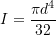### Review of single and multi-degree of freedom (mdof) systems: Equivalent spring constants

One of the components we need in these equations of motion is the spring constant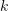. We can often find this for a system using static techniques. This is easily generalized for MDOF systems.

For SDOF systems, we can imagine the static response of the system using one of the approaches:

1.Apply a unit force (moment) to the mass (inertia) in the positive direction of motion then calculate the displacement that occurs (flexibility approach).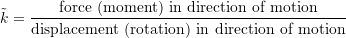2.Apply a unit displacement (rotation) to the mass (inertia) in the positive direction of motion then calculate the force (moment) required to maintain it (stiffness approach).It depends on the situation to determine the best one to use. Generally, for a series situation, use flexibility, while for parallel, use stiffness.

#### Springs in Series

Apply unit load, and calculate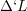.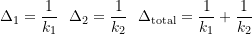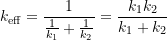#### Springs in Parallel

Apply unit deflection, and calculate load.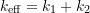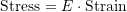Therefore: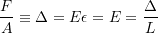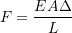Therefore: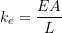#### Inclined Axial Spring

Apply a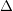in the direction of motion.

Therefore, force in spring when extended is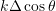, and thus: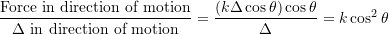#### Example 1

Stiffness
Apply unit deflection, and calculate total load.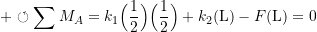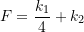Therefore: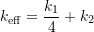If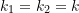, then: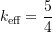#### Example 2

Flexibility
Apply unit load, and calculate total deflection.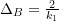, therefore: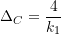Therefore :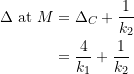Thus: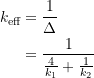If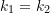, then: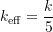#### Equivalent Spring Constant Equations

1.axial springs in parallel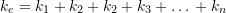2.axial springs in series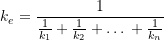3.Springs in parallel and series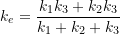4.Inclined axial spring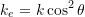5.Rotating bar with spring support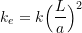6.Rigid bar supported on two springs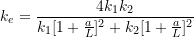7.Rigid bar supported on three springs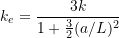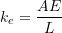Where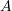is the cross-sectional area, and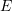is the elastic modulus.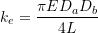10.Axially helical spring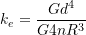Whereis the active number of turns, and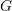is the elastic shear modulus.

11.Torsion of a uniform shaft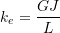Where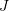is the torsional constant of cross section (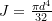).

12.Torsion of a tapered circular shaft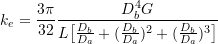13.Spiral torsional spring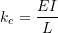Whereis Young’s modulus.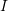is the moment of inertia of cross-sectional area, and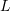is the total length of the spiral.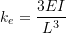15.Simply supported bean, load at midspan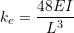16.Simply supported bean, load anywhere between supports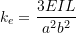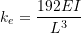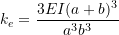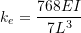20.Propped cantilever, load at free end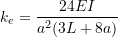#### Table 2-1

###### Fixed-fixed beam*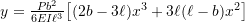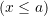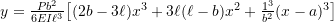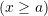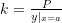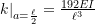###### Fixed-pinned beam with overhang*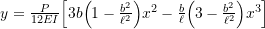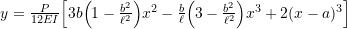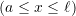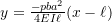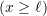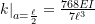###### Fixed-pinned beam with overhang (P at x = l + a)*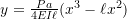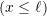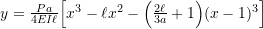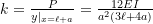###### Pinned-pinned beam with overhang*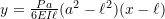###### Pinned-pinned beam with overhang (P at x = l + a)*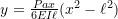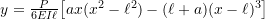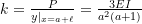###### Fixed-fixed beam with lateral displacement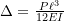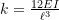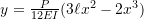* – Axial extensions due to axial end constraints considered negligible

#### Torsional Oscillations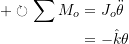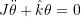Let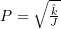. From the strength of the material: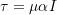Where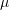is the modulus of rigidity,is the angle of twist/unit length andis the polar second moment of inertia. Therefore: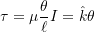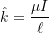For soild circular cross sections: